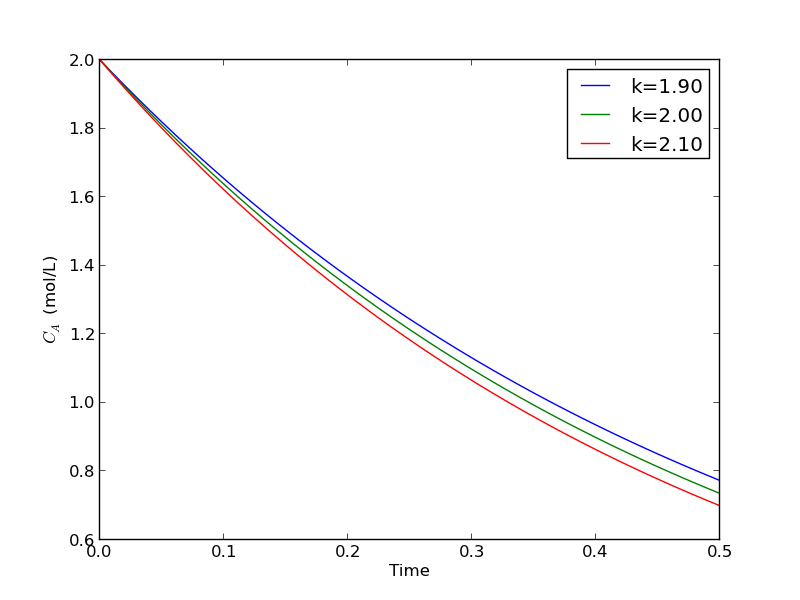Solving parameterized ODEs over and over conveniently

| categories: ode | tags: reaction engineering | View Comments

Matlab post Sometimes we have an ODE that depends on a parameter, and we want to solve the ODE for several parameter values. It is inconvenient to write an ode function for each parameter case. Here we examine a convenient way to solve this problem; we pass the parameter to the ODE at runtime. We consider the following ODE:

$$\frac{dCa}{dt} = -k Ca(t)$$

where $$k$$ is a parameter, and we want to solve the equation for a couple of values of $$k$$ to test the sensitivity of the solution on the parameter. Our question is, given $$Ca(t=0)=2$$, how long does it take to get $$Ca = 1$$, and how sensitive is the answer to small variations in $$k$$?

import numpy as np
from scipy.integrate import odeint
import matplotlib.pyplot as plt

def myode(Ca, t, k):
'ODE definition'

tspan = np.linspace(0, 0.5)
k0 = 2
Ca0 = 2

plt.figure(); plt.clf()

for k in [0.95 * k0, k0, 1.05 * k0]:
sol = odeint(myode, Ca0, tspan, args=(k,))
plt.plot(tspan, sol, label='k={0:1.2f}'.format(k))
print 'At t=0.5 Ca = {0:1.2f} mol/L'.format(sol[-1])

plt.legend(loc='best')
plt.xlabel('Time')
plt.ylabel('$C_A$ (mol/L)')
plt.savefig('images/parameterized-ode1.png')

At t=0.5 Ca = 0.77 mol/L
At t=0.5 Ca = 0.74 mol/L
At t=0.5 Ca = 0.70 mol/LYou can see there are some variations in the concentration at t = 0.5. You could over or underestimate the concentration if you have the wrong estimate of $k$! You have to use some judgement here to decide how long to run the reaction to ensure a target goal is met.